Anzeige

# C Programming Unit-2

10. Aug 2019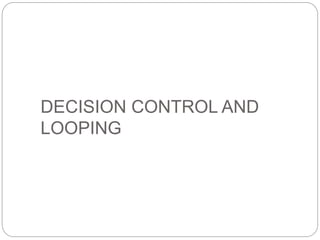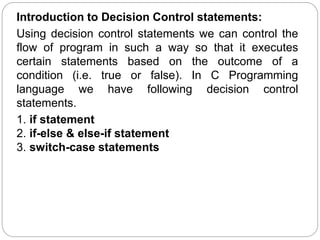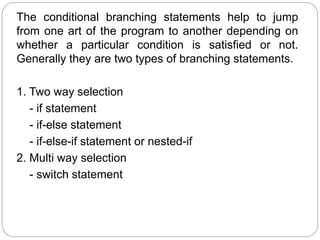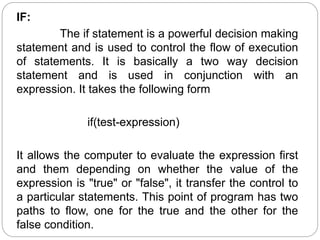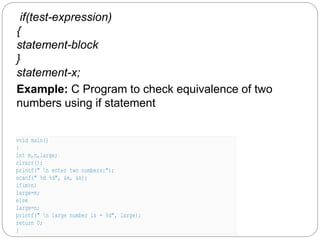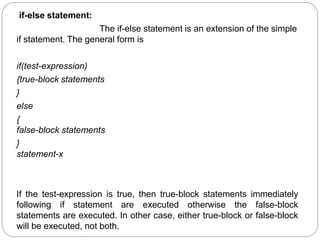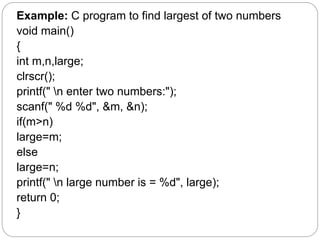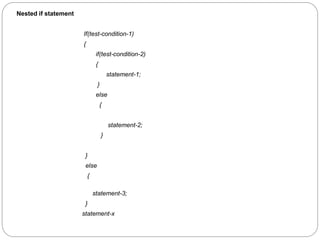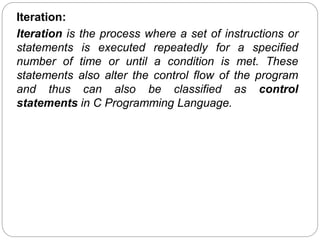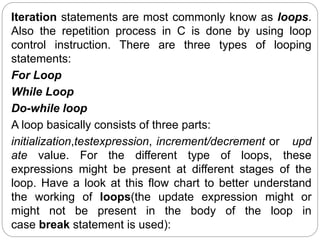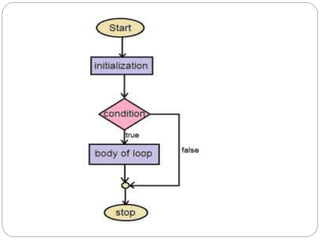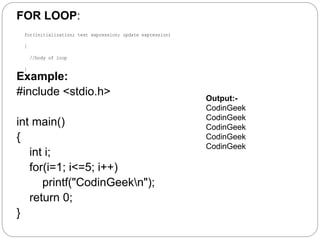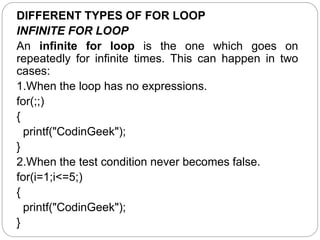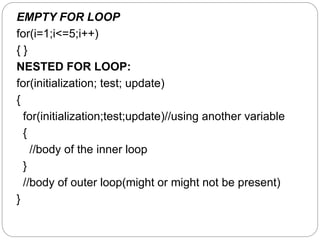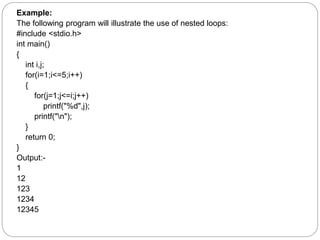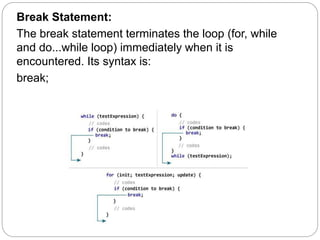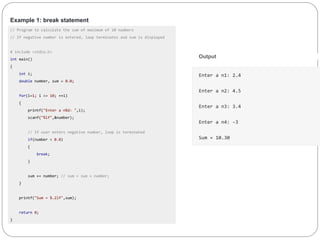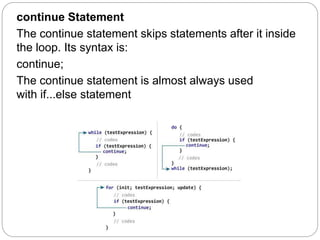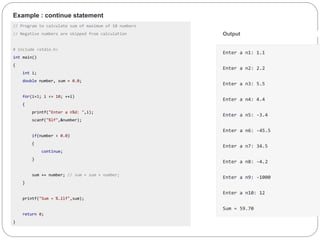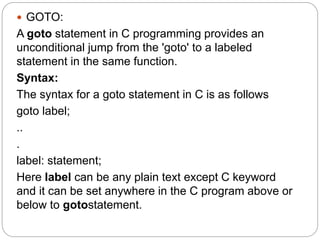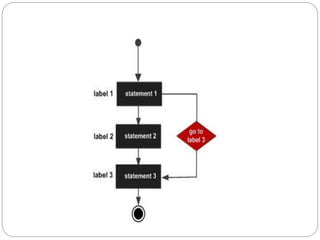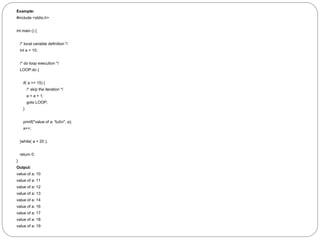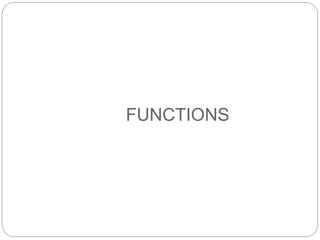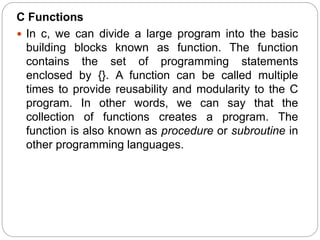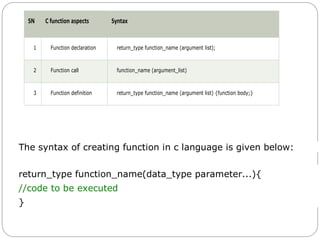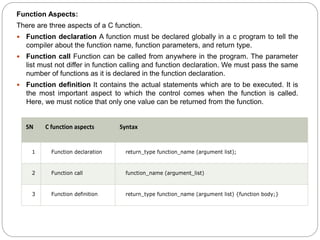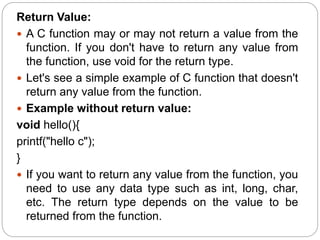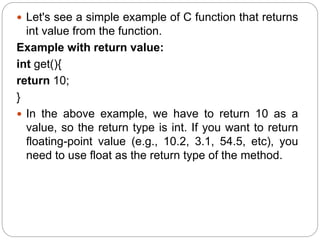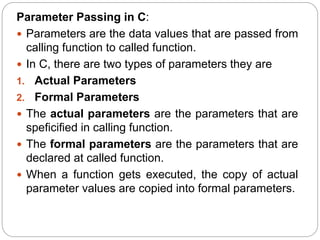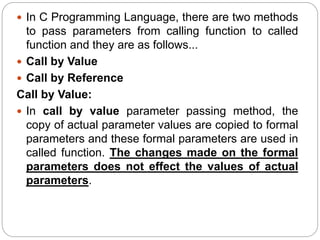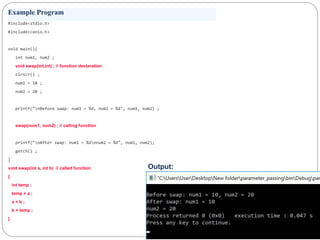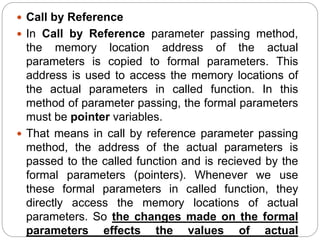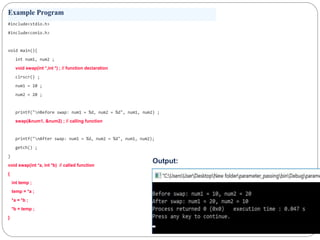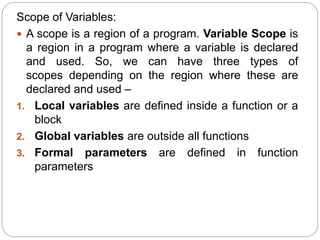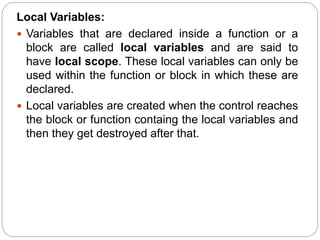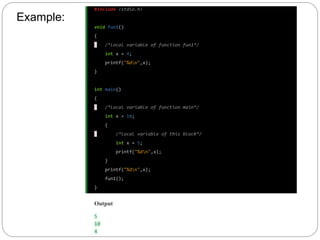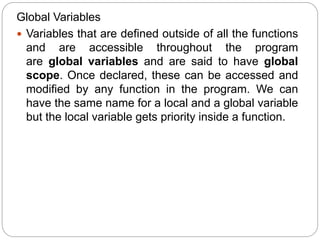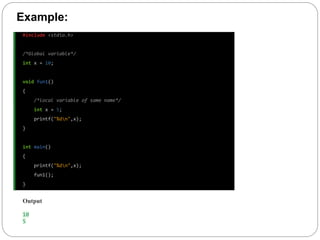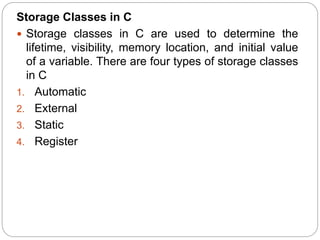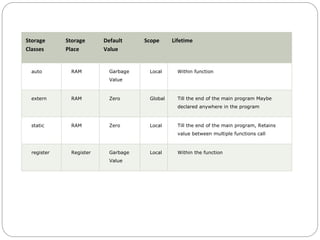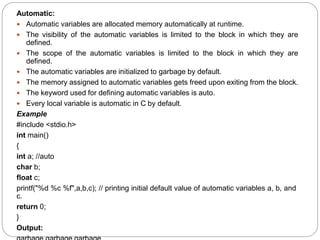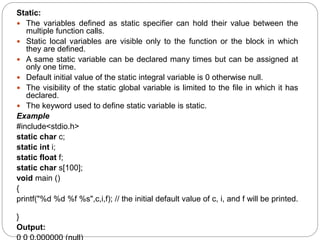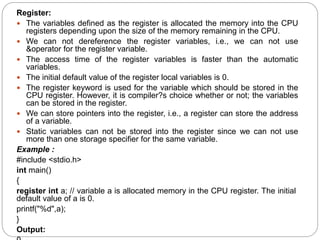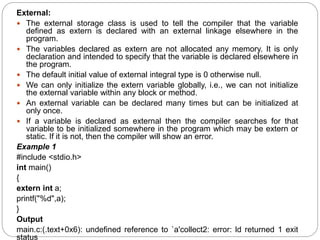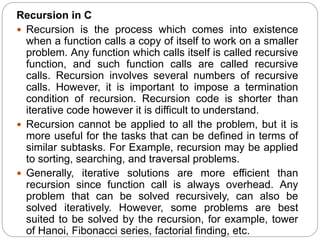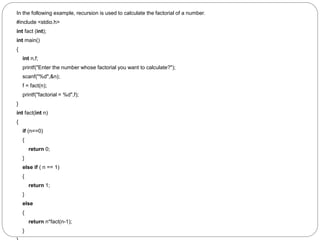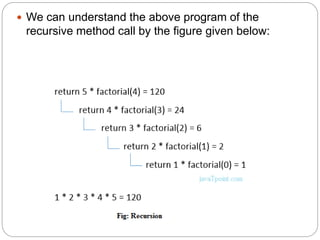1 von 47
Anzeige

### C Programming Unit-2

1. DECISION CONTROL AND LOOPING
2. Introduction to Decision Control statements: Using decision control statements we can control the flow of program in such a way so that it executes certain statements based on the outcome of a condition (i.e. true or false). In C Programming language we have following decision control statements. 1. if statement 2. if-else & else-if statement 3. switch-case statements
3. The conditional branching statements help to jump from one art of the program to another depending on whether a particular condition is satisfied or not. Generally they are two types of branching statements. 1. Two way selection - if statement - if-else statement - if-else-if statement or nested-if 2. Multi way selection - switch statement
4. IF: The if statement is a powerful decision making statement and is used to control the flow of execution of statements. It is basically a two way decision statement and is used in conjunction with an expression. It takes the following form if(test-expression) It allows the computer to evaluate the expression first and them depending on whether the value of the expression is "true" or "false", it transfer the control to a particular statements. This point of program has two paths to flow, one for the true and the other for the false condition.
5. if(test-expression) { statement-block } statement-x; Example: C Program to check equivalence of two numbers using if statement void main() { int m,n,large; clrscr(); printf(" n enter two numbers:"); scanf(" %d %d", &m, &n); if(m>n) large=m; else large=n; printf(" n large number is = %d", large); return 0; }
6. if-else statement: The if-else statement is an extension of the simple if statement. The general form is if(test-expression) {true-block statements } else { false-block statements } statement-x If the test-expression is true, then true-block statements immediately following if statement are executed otherwise the false-block statements are executed. In other case, either true-block or false-block will be executed, not both.
7. Example: C program to find largest of two numbers void main() { int m,n,large; clrscr(); printf(" n enter two numbers:"); scanf(" %d %d", &m, &n); if(m>n) large=m; else large=n; printf(" n large number is = %d", large); return 0; }
8. Nested if statement If(test-condition-1) { if(test-condition-2) { statement-1; } else { statement-2; } } else { statement-3; } statement-x
9. Iteration: Iteration is the process where a set of instructions or statements is executed repeatedly for a specified number of time or until a condition is met. These statements also alter the control flow of the program and thus can also be classified as control statements in C Programming Language.
10. Iteration statements are most commonly know as loops. Also the repetition process in C is done by using loop control instruction. There are three types of looping statements: For Loop While Loop Do-while loop A loop basically consists of three parts: initialization,testexpression, increment/decrement or upd ate value. For the different type of loops, these expressions might be present at different stages of the loop. Have a look at this flow chart to better understand the working of loops(the update expression might or might not be present in the body of the loop in case break statement is used):
11. FOR LOOP: Example: #include <stdio.h> int main() { int i; for(i=1; i<=5; i++) printf("CodinGeekn"); return 0; } for(initialization; test expression; update expression) { //body of loop } Output:- CodinGeek CodinGeek CodinGeek CodinGeek CodinGeek
12. DIFFERENT TYPES OF FOR LOOP INFINITE FOR LOOP An infinite for loop is the one which goes on repeatedly for infinite times. This can happen in two cases: 1.When the loop has no expressions. for(;;) { printf("CodinGeek"); } 2.When the test condition never becomes false. for(i=1;i<=5;) { printf("CodinGeek"); }
13. EMPTY FOR LOOP for(i=1;i<=5;i++) { } NESTED FOR LOOP: for(initialization; test; update) { for(initialization;test;update)//using another variable { //body of the inner loop } //body of outer loop(might or might not be present) }
14. Example: The following program will illustrate the use of nested loops: #include <stdio.h> int main() { int i,j; for(i=1;i<=5;i++) { for(j=1;j<=i;j++) printf("%d",j); printf("n"); } return 0; } Output:- 1 12 123 1234 12345
15. Break Statement: The break statement terminates the loop (for, while and do...while loop) immediately when it is encountered. Its syntax is: break;
16. Example 1: break statement // Program to calculate the sum of maximum of 10 numbers // If negative number is entered, loop terminates and sum is displayed # include <stdio.h> int main() { int i; double number, sum = 0.0; for(i=1; i <= 10; ++i) { printf("Enter a n%d: ",i); scanf("%lf",&number); // If user enters negative number, loop is terminated if(number < 0.0) { break; } sum += number; // sum = sum + number; } printf("Sum = %.2lf",sum); return 0; } Output Enter a n1: 2.4 Enter a n2: 4.5 Enter a n3: 3.4 Enter a n4: -3 Sum = 10.30
17. continue Statement The continue statement skips statements after it inside the loop. Its syntax is: continue; The continue statement is almost always used with if...else statement
18. Example : continue statement // Program to calculate sum of maximum of 10 numbers // Negative numbers are skipped from calculation # include <stdio.h> int main() { int i; double number, sum = 0.0; for(i=1; i <= 10; ++i) { printf("Enter a n%d: ",i); scanf("%lf",&number); if(number < 0.0) { continue; } sum += number; // sum = sum + number; } printf("Sum = %.2lf",sum); return 0; } Output Enter a n1: 1.1 Enter a n2: 2.2 Enter a n3: 5.5 Enter a n4: 4.4 Enter a n5: -3.4 Enter a n6: -45.5 Enter a n7: 34.5 Enter a n8: -4.2 Enter a n9: -1000 Enter a n10: 12 Sum = 59.70
19.  GOTO: A goto statement in C programming provides an unconditional jump from the 'goto' to a labeled statement in the same function. Syntax: The syntax for a goto statement in C is as follows goto label; .. . label: statement; Here label can be any plain text except C keyword and it can be set anywhere in the C program above or below to gotostatement.
20. Example: #include <stdio.h> int main () { /* local variable definition */ int a = 10; /* do loop execution */ LOOP:do { if( a == 15) { /* skip the iteration */ a = a + 1; goto LOOP; } printf("value of a: %dn", a); a++; }while( a < 20 ); return 0; } Output: value of a: 10 value of a: 11 value of a: 12 value of a: 13 value of a: 14 value of a: 16 value of a: 17 value of a: 18 value of a: 19
21. FUNCTIONS
22. C Functions  In c, we can divide a large program into the basic building blocks known as function. The function contains the set of programming statements enclosed by {}. A function can be called multiple times to provide reusability and modularity to the C program. In other words, we can say that the collection of functions creates a program. The function is also known as procedure or subroutine in other programming languages.
23. SN C function aspects Syntax 1 Function declaration return_type function_name (argument list); 2 Function call function_name (argument_list) 3 Function definition return_type function_name (argument list) {function body;} The syntax of creating function in c language is given below: 1. return_type function_name(data_type parameter...){ 2. //code to be executed 3. }
24. Function Aspects: There are three aspects of a C function.  Function declaration A function must be declared globally in a c program to tell the compiler about the function name, function parameters, and return type.  Function call Function can be called from anywhere in the program. The parameter list must not differ in function calling and function declaration. We must pass the same number of functions as it is declared in the function declaration.  Function definition It contains the actual statements which are to be executed. It is the most important aspect to which the control comes when the function is called. Here, we must notice that only one value can be returned from the function. SN C function aspects Syntax 1 Function declaration return_type function_name (argument list); 2 Function call function_name (argument_list) 3 Function definition return_type function_name (argument list) {function body;}
25. Return Value:  A C function may or may not return a value from the function. If you don't have to return any value from the function, use void for the return type.  Let's see a simple example of C function that doesn't return any value from the function.  Example without return value: void hello(){ printf("hello c"); }  If you want to return any value from the function, you need to use any data type such as int, long, char, etc. The return type depends on the value to be returned from the function.
26.  Let's see a simple example of C function that returns int value from the function. Example with return value: int get(){ return 10; }  In the above example, we have to return 10 as a value, so the return type is int. If you want to return floating-point value (e.g., 10.2, 3.1, 54.5, etc), you need to use float as the return type of the method.
27. Parameter Passing in C:  Parameters are the data values that are passed from calling function to called function.  In C, there are two types of parameters they are 1. Actual Parameters 2. Formal Parameters  The actual parameters are the parameters that are speficified in calling function.  The formal parameters are the parameters that are declared at called function.  When a function gets executed, the copy of actual parameter values are copied into formal parameters.
28.  In C Programming Language, there are two methods to pass parameters from calling function to called function and they are as follows...  Call by Value  Call by Reference Call by Value:  In call by value parameter passing method, the copy of actual parameter values are copied to formal parameters and these formal parameters are used in called function. The changes made on the formal parameters does not effect the values of actual parameters.
29. Example Program #include<stdio.h> #include<conio.h> void main(){ int num1, num2 ; void swap(int,int) ; // function declaration clrscr() ; num1 = 10 ; num2 = 20 ; printf("nBefore swap: num1 = %d, num2 = %d", num1, num2) ; swap(num1, num2) ; // calling function printf("nAfter swap: num1 = %dnnum2 = %d", num1, num2); getch() ; } void swap(int a, int b) // called function { int temp ; temp = a ; a = b ; b = temp ; } Output:
30.  Call by Reference  In Call by Reference parameter passing method, the memory location address of the actual parameters is copied to formal parameters. This address is used to access the memory locations of the actual parameters in called function. In this method of parameter passing, the formal parameters must be pointer variables.  That means in call by reference parameter passing method, the address of the actual parameters is passed to the called function and is recieved by the formal parameters (pointers). Whenever we use these formal parameters in called function, they directly access the memory locations of actual parameters. So the changes made on the formal parameters effects the values of actual
31. Example Program #include<stdio.h> #include<conio.h> void main(){ int num1, num2 ; void swap(int *,int *) ; // function declaration clrscr() ; num1 = 10 ; num2 = 20 ; printf("nBefore swap: num1 = %d, num2 = %d", num1, num2) ; swap(&num1, &num2) ; // calling function printf("nAfter swap: num1 = %d, num2 = %d", num1, num2); getch() ; } void swap(int *a, int *b) // called function { int temp ; temp = *a ; *a = *b ; *b = temp ; } Output:
32. Scope of Variables:  A scope is a region of a program. Variable Scope is a region in a program where a variable is declared and used. So, we can have three types of scopes depending on the region where these are declared and used – 1. Local variables are defined inside a function or a block 2. Global variables are outside all functions 3. Formal parameters are defined in function parameters
33. Local Variables:  Variables that are declared inside a function or a block are called local variables and are said to have local scope. These local variables can only be used within the function or block in which these are declared.  Local variables are created when the control reaches the block or function containg the local variables and then they get destroyed after that.
34. Example: #include <stdio.h> void fun1() { /*local variable of function fun1*/ int x = 4; printf("%dn",x); } int main() { /*local variable of function main*/ int x = 10; { /*local variable of this block*/ int x = 5; printf("%dn",x); } printf("%dn",x); fun1(); } Output 5 10 4
35. Global Variables  Variables that are defined outside of all the functions and are accessible throughout the program are global variables and are said to have global scope. Once declared, these can be accessed and modified by any function in the program. We can have the same name for a local and a global variable but the local variable gets priority inside a function.
36. Example: #include <stdio.h> /*Global variable*/ int x = 10; void fun1() { /*local variable of same name*/ int x = 5; printf("%dn",x); } int main() { printf("%dn",x); fun1(); } Output 10 5
37. Storage Classes in C  Storage classes in C are used to determine the lifetime, visibility, memory location, and initial value of a variable. There are four types of storage classes in C 1. Automatic 2. External 3. Static 4. Register
38. Storage Classes Storage Place Default Value Scope Lifetime auto RAM Garbage Value Local Within function extern RAM Zero Global Till the end of the main program Maybe declared anywhere in the program static RAM Zero Local Till the end of the main program, Retains value between multiple functions call register Register Garbage Value Local Within the function
39. Automatic:  Automatic variables are allocated memory automatically at runtime.  The visibility of the automatic variables is limited to the block in which they are defined.  The scope of the automatic variables is limited to the block in which they are defined.  The automatic variables are initialized to garbage by default.  The memory assigned to automatic variables gets freed upon exiting from the block.  The keyword used for defining automatic variables is auto.  Every local variable is automatic in C by default. Example #include <stdio.h> int main() { int a; //auto char b; float c; printf("%d %c %f",a,b,c); // printing initial default value of automatic variables a, b, and c. return 0; } Output:
40. Static:  The variables defined as static specifier can hold their value between the multiple function calls.  Static local variables are visible only to the function or the block in which they are defined.  A same static variable can be declared many times but can be assigned at only one time.  Default initial value of the static integral variable is 0 otherwise null.  The visibility of the static global variable is limited to the file in which it has declared.  The keyword used to define static variable is static. Example #include<stdio.h> static char c; static int i; static float f; static char s; void main () { printf("%d %d %f %s",c,i,f); // the initial default value of c, i, and f will be printed. } Output:
41. Register:  The variables defined as the register is allocated the memory into the CPU registers depending upon the size of the memory remaining in the CPU.  We can not dereference the register variables, i.e., we can not use &operator for the register variable.  The access time of the register variables is faster than the automatic variables.  The initial default value of the register local variables is 0.  The register keyword is used for the variable which should be stored in the CPU register. However, it is compiler?s choice whether or not; the variables can be stored in the register.  We can store pointers into the register, i.e., a register can store the address of a variable.  Static variables can not be stored into the register since we can not use more than one storage specifier for the same variable. Example : #include <stdio.h> int main() { register int a; // variable a is allocated memory in the CPU register. The initial default value of a is 0. printf("%d",a); } Output:
42. External:  The external storage class is used to tell the compiler that the variable defined as extern is declared with an external linkage elsewhere in the program.  The variables declared as extern are not allocated any memory. It is only declaration and intended to specify that the variable is declared elsewhere in the program.  The default initial value of external integral type is 0 otherwise null.  We can only initialize the extern variable globally, i.e., we can not initialize the external variable within any block or method.  An external variable can be declared many times but can be initialized at only once.  If a variable is declared as external then the compiler searches for that variable to be initialized somewhere in the program which may be extern or static. If it is not, then the compiler will show an error. Example 1 #include <stdio.h> int main() { extern int a; printf("%d",a); } Output main.c:(.text+0x6): undefined reference to `a'collect2: error: ld returned 1 exit status
43. Recursion in C  Recursion is the process which comes into existence when a function calls a copy of itself to work on a smaller problem. Any function which calls itself is called recursive function, and such function calls are called recursive calls. Recursion involves several numbers of recursive calls. However, it is important to impose a termination condition of recursion. Recursion code is shorter than iterative code however it is difficult to understand.  Recursion cannot be applied to all the problem, but it is more useful for the tasks that can be defined in terms of similar subtasks. For Example, recursion may be applied to sorting, searching, and traversal problems.  Generally, iterative solutions are more efficient than recursion since function call is always overhead. Any problem that can be solved recursively, can also be solved iteratively. However, some problems are best suited to be solved by the recursion, for example, tower of Hanoi, Fibonacci series, factorial finding, etc.
44. In the following example, recursion is used to calculate the factorial of a number. #include <stdio.h> int fact (int); int main() { int n,f; printf("Enter the number whose factorial you want to calculate?"); scanf("%d",&n); f = fact(n); printf("factorial = %d",f); } int fact(int n) { if (n==0) { return 0; } else if ( n == 1) { return 1; } else { return n*fact(n-1); }
45.  We can understand the above program of the recursive method call by the figure given below:
Anzeige